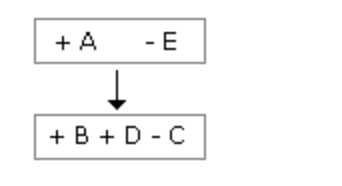## Blood Relations

``` (adsbygoogle = window.adsbygoogle || []).push({}); ```

``` (adsbygoogle = window.adsbygoogle || []).push({}); ```

## Blood Relations Introduction

The questions which are asked in this section depend upon Relation. You should have a sound knowledge of the blood relation in order to solve the questions.

To remember easily the relations may be divided into two sides as given below:

1: Relations of Paternal side:

Father’s father → Grandfather

Father’s mother → Grandmother

Father’s brother → Uncle

Father’s sister → Aunt

Children of uncle → Cousin

Wife of uncle → Aunt

Children of aunt → Cousin

Husband of aunt → Uncle

2: Relations of Maternal side:

Mother’s father → Maternal grandfather

Mother’s mother → Maternal grandmother

Mother’s brother Maternal uncle

Mother’s sister → Aunt

Children of maternal uncle → Cousin

Wife of maternal uncle → Maternal aunt

Relations from one generation to next:

Different types of questions with explanation:

### Type 1

If A + B means A is the mother of B; A x B means A is the father of B; A \$ B means A is the brother of B and A @ B means A is the sister of B then which of the following means P is the son of Q?

(A) Q + R @ P @ N       (B) Q + R * P @ N

(C) Q x R \$ P @ N        (D) Q x R \$ P \$ N

Solution: (D)

Q x R = Q is the mother of R [-Q, ±R]

R \$ P = R is the brother of P [+ R, ±P]

P \$ N = P is the brother of N [+ P, ±N]

Therefore P is the son of Q.

### Type 2

A has 3 children. B is the brother of C and C is the sister of D, E who is the wife of A is the mother of D. There is only one daughter of the husband of E. what is the relation between D and B?

Solution: With the chart

Therefore, D is a boy because there is only one daughter of E.Hence, B is the brother of D.

### Type 3

Pointing to a photograph, Rekha says to Lalli, “The girl in the photo is the second daughter of the wife of only son of the grandmother of my younger sister.” How this girl of photograph is related to Rekha?

Solution:

Grandmother of younger sister of Rekha → Grandmother of Rekha

Wife of only son of grandmother → Mother of Rekha

Younger daughter of the mother → Younger sister.

Note: While solving the question (+) can be used for male and (-) can be used for female.

``` (adsbygoogle = window.adsbygoogle || []).push({}); ```

Jobs By Batch
Jobs By Location
Jobs By Degree
Jobs By Branch
IT Jobs
Internships
Govt. Jobs

``` (adsbygoogle = window.adsbygoogle || []).push({}); ```

• ##### Replicon off campus hiring drive 2022 | Product Support Trainee | BE/B.Tech/BCA/B.Sc | 3.3LPA

``` (adsbygoogle = window.adsbygoogle || []).push({}); ```

• ##### Original Questions of IT Companies| All Topics

``` (adsbygoogle = window.adsbygoogle || []).push({}); ```